Remarks: By default, we show e-Lecture Mode for first time (or non logged-in) visitor.

X Esc

1. 比较非比较策略，
2. 迭代与递归实现，
3. 分而治之范式（这个这个），
4. 最佳/最差/平均情况时间复杂性分析，
5. 随机算法

Pro-tip: Since you are not logged-in, you may be a first time visitor who are not aware of the following keyboard shortcuts to navigate this e-Lecture mode: [PageDown] to advance to the next slide, [PageUp] to go back to the previous slide, [Esc] to toggle between this e-Lecture mode and exploration mode.

X Esc

1. 在数组 A 中搜索特定值 v
2. 查找（静态）数组 A 中的最小/最大/第 k 个最小/最大值，
3. 测试唯一性并删除数组 A 中的重复项，
4. 计算特定值 v 在数组 A 中出现多少次，
5. 设置数组 A 和另一个排序数组 B 之间的交集/联合，
6. 寻找一个目标对 x∈Ay∈A，使得 x + y 等于目标 z 等。

Another pro-tip: We designed this visualization and this e-Lecture mode to look good on 1366x768 resolution or larger (typical modern laptop resolution in 2017). We recommend using Google Chrome to access VisuAlgo. Go to full screen mode (F11) to enjoy this setup. However, you can use zoom-in (Ctrl +) or zoom-out (Ctrl -) to calibrate this.

X Esc

X Esc

1. 完全随机的
2. 随机排序（按升序/降序排列），
3. 随机但几乎排序（按升序/降序排列）或
4. 完全由你自己定义。

X Esc

X Esc

X Esc

X Esc

1. 基于比较的排序算法:
1. BUB - 冒泡排序,
2. SEL - 选择排序,
3. INS - 插入排序,
4. MER - 归并排序 (递归实现),
5. QUI - 快速排序 (递归实现),
6. R-Q - 随机快速排序 (递归实现).
2. 不基于比较的排序算法:
1. COU - 计数排序,
X Esc

X Esc

Before we start with the discussion of various sorting algorithms, it may be a good idea to discuss the basics of asymptotic algorithm analysis, so that you can follow the discussions of the various O(N^2), O(N log N), and special O(N) sorting algorithms later.

This section can be skipped if you already know this topic.

X Esc

You need to already understand/remember all these:
-. Logarithm and Exponentiation, e.g., log2(1024) = 10, 210 = 1024
-. Arithmetic progression, e.g., 1+2+3+4+…+10 = 10*11/2 = 55
-. Geometric progression, e.g., 1+2+4+8+..+1024 = 1*(1-211)/(1-2) = 2047
-. Linear/Quadratic/Cubic function, e.g., f1(x) = x+2, f2(x) = x2+x-1, f3(x) = x3+2x2-x+7
-. Ceiling, Floor, and Absolute function, e.g., ceil(3.1) = 4, floor(3.1) = 3, abs(-7) = 7

X Esc

Analysis of Algorithm is a process to evaluate rigorously the resources (time and space) needed by an algorithm and represent the result of the evaluation with a (simple) formula.

The time/space requirement of an algorithm is also called the time/space complexity of the algorithm, respectively.

For this module, we focus more on time requirement of various sorting algorithms.

X Esc

We can measure the actual running time of a program by using wall clock time or by inserting timing-measurement code into our program, e.g., see the code shown in SpeedTest.cpp|java|py.

However, actual running time is not meaningful when comparing two algorithms as they are possibly coded in different languages, using different data sets, or running on different computers.

X Esc

Instead of measuring the actual timing, we count the # of operations (arithmetic, assignment, comparison, etc). This is a way to assess its efficiency as an algorithm's execution time is correlated to the # of operations that it requires.

See the code shown in SpeedTest.cpp|java|py and the comments (especially on how to get the final value of variable counter).

Knowing the (precise) number of operations required by the algorithm, we can state something like this: Algorithm X takes 2n2 + 100n operations to solve problem of size n.

X Esc

If the time t needed for one operation is known, then we can state that algorithm X takes (2n2 + 100n)t time units to solve problem of size n.

However, time t is dependent on the factors mentioned earlier, e.g., different languages, compilers and computers, etc.

Therefore, instead of tying the analysis to actual time t, we can state that algorithm X takes time that is proportional to 2n2 + 100n to solving problem of size n.

X Esc

Asymptotic analysis is an analysis of algorithms that focuses on analyzing problems of large input size n, considers only the leading term of the formula, and ignores the coefficient of the leading term.

We choose the leading term because the lower order terms contribute lesser to the overall cost as the input grows larger, e.g., for f(n) = 2n2 + 100n, we have:
f(1000) = 2*10002 + 100*1000 = 2.1M, vs
f(100000) = 2*1000002 + 100*100000 = 20010M.
(notice that the lower order term 100n has lesser contribution).

X Esc

Suppose two algorithms have 2n2 and 30n2 as the leading terms, respectively.

Although actual time will be different due to the different constants, the growth rates of the running time are the same.

Compared with another algorithm with leading term of n3, the difference in growth rate is a much more dominating factor.

Hence, we can drop the coefficient of leading term when studying algorithm complexity.

X Esc

If algorithm A requires time proportional to f(n), we say that algorithm A is of the order of f(n).

We write that algorithm A has time complexity of O(f(n)), where f(n) is the growth rate function for algorithm A.

X Esc

Mathematically, an algorithm A is of O(f(n)) if there exist a constant k and a positive integer n0 such that algorithm A requires no more than k*f(n) time units to solve a problem of size n ≥ n0, i.e., when the problem size is larger than n0 algorithm A is (always) bounded from above by this simple formula k*f(n).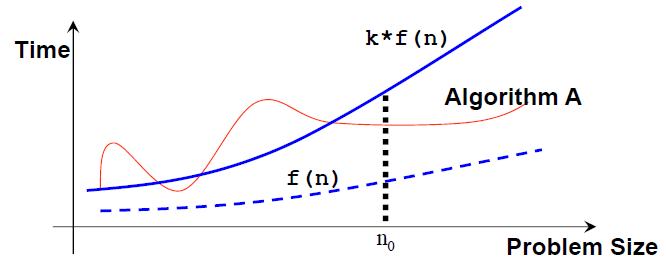Note that: n0 and k are not unique and there can be many possible valid f(n).

X Esc

In asymptotic analysis, a formula can be simplified to a single term with coefficient 1.

Such a term is called a growth term (rate of growth, order of growth, order of magnitude).

The most common growth terms can be ordered from fastest to slowest as follows
Note that many others are not shown (also see the visualization in the next slide):
O(1)/constant time < O(log n)/logarithmic time < O(n)/linear time <
O(n log n)/quasilinear time < O(n2)/quadratic time < O(n3)/cubic time <
O(2n)/exponential time < O(n!)/also-exponential time < ...

X Esc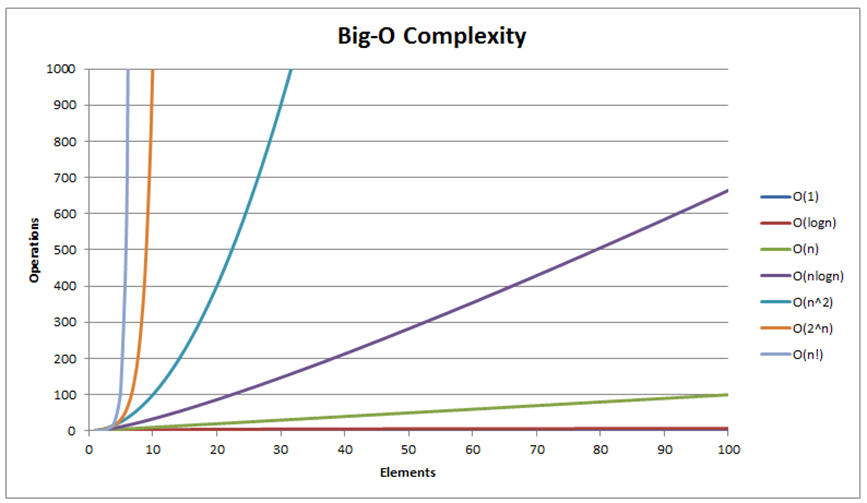We will see three different growth rates O(n2), O(n log n), and O(n) throughout the remainder of this sorting module.

X Esc

1. 如果元素大小关系不正确，交换这两个数（在本例中为a> b），
2. 比较一对相邻元素（a，b），
3. 重复步骤1和2，直到我们到达数组的末尾（最后一对是第（N-2）和（N-1）项，因为我们的数组从零开始）
4. 到目前为止，最大的元素将在最后的位置。 然后我们将N减少1，并重复步骤1，直到N = 1。

X Esc

（标准）Bubble Sort中有两个嵌套循环。

1. 当 i = 0，（N-1）次迭代（比较和可能交换）时。
2. 当 i = 1，（N-2）次迭代时，...
3. 当 i =（N-2）时，1次迭代,
4. 当 i=（N-1），0迭代.

X Esc

X Esc

e-Lecture: The content of this slide is hidden and only available for legitimate CS lecturer worldwide. Drop an email to visualgo.info at gmail dot com if you want to activate this CS lecturer-only feature and you are really a CS lecturer (show your University staff profile).

X Esc

1. [L ... N-1] 范围内找出最小项目 X 的位置，
2. 用第 L 项交换X，
3. 将下限 L 增加1并重复步骤1直到 L = N-2

X Esc

`void selectionSort(int a[], int N) {  for (int L = 0; L <= N-2; ++L) { // O(N)    int X = min_element(a+L, a+N) - a; // O(N)    swap(a[X], a[L]); // O(1), X may be equal to L (no actual swap)  }}`

Total: O(N2) — To be precise, it is similar to Bubble Sort analysis.

X Esc

Quiz: How many (real) swaps are required to sort [29, 10, 14, 37, 13] by Selection Sort?

4
3
2
1
X Esc

1. 从你手中的一张牌开始，
2. 选择下一张卡并将其插入到正确的排序顺序中，
3. 对所有的卡重复上一步。

X Esc

`void insertionSort(int a[], int N) {  for (int i = 1; i < N; ++i) { // O(N)    int X = a[i]; // X is the item to be inserted    int j = i-1;    for (; j >= 0 && a[j] > X; --j) // can be fast or slow      a[j+1] = a[j]; // make a place for X    a[j+1] = X; // index j+1 is the insertion point  }}`
X Esc

1. 在最好的情况下，数组已经排序并且（a [j]> X）总是为假所以不需要移位数据，并且内部循环运行在O（1），
2. 在最坏的情况下，数组被反向排序并且（a [j]> X）始终为真插入始终发生在数组的前端，并且内部循环以O（N）运行。

X Esc

Quiz: What is the complexity of Insertion Sort on any input array?

O(N)
O(N^2)
O(N log N)
O(1)

X Esc

1. 归并排序
2. 快速排序和它的随机版本（只有一个变化）。

PS：尽管如此，快速排序（Quick Sort）的非随机版本在 O(N2中运行。
X Esc

1. 将每对单个元素（默认情况下，已排序）归并为2个元素的有序数组，
2. 将2个元素的每对有序数组归并成4个元素的有序数组，重复这个过程......，
3. 最后一步：归并2个N / 2元素的排序数组（为了简化讨论，我们假设N是偶数）以获得完全排序的N个元素数组。

X Esc

X Esc

`void merge(int a[], int low, int mid, int high) {  // subarray1 = a[low..mid], subarray2 = a[mid+1..high], both sorted  int N = high-low+1;  int b[N]; // 讨论: 为什么我们需要一个临时的数组 b?  int left = low, right = mid+1, bIdx = 0;  while (left <= mid && right <= high) // 归并    b[bIdx++] = (a[left] <= a[right]) ? a[left++] : a[right++];  while (left <= mid) b[bIdx++] = a[left++]; // leftover, if any  while (right <= high) b[bIdx++] = a[right++]; // leftover, if any  for (int k = 0; k < N; k++) a[low+k] = b[k]; // copy back}`

X Esc

1. 划分步骤：将大的原始问题划分成较小的子问题并递归地解决较小的子问题，
2. 解决步骤：结合较小的子问题的结果来产生较大的原始问题的结果。
X Esc

X Esc

`void mergeSort(int a[], int low, int high) {  // 要排序的数组是 a[low..high]  if (low < high) { // base case: low >= high (0 or 1 item)    int mid = (low+high) / 2;	    mergeSort(a, low  , mid ); // 分成一半    mergeSort(a, mid+1, high); // 递归地将它们排序    merge(a, low, mid, high); // 解决: 归并子程序  }}`
X Esc

X Esc

X Esc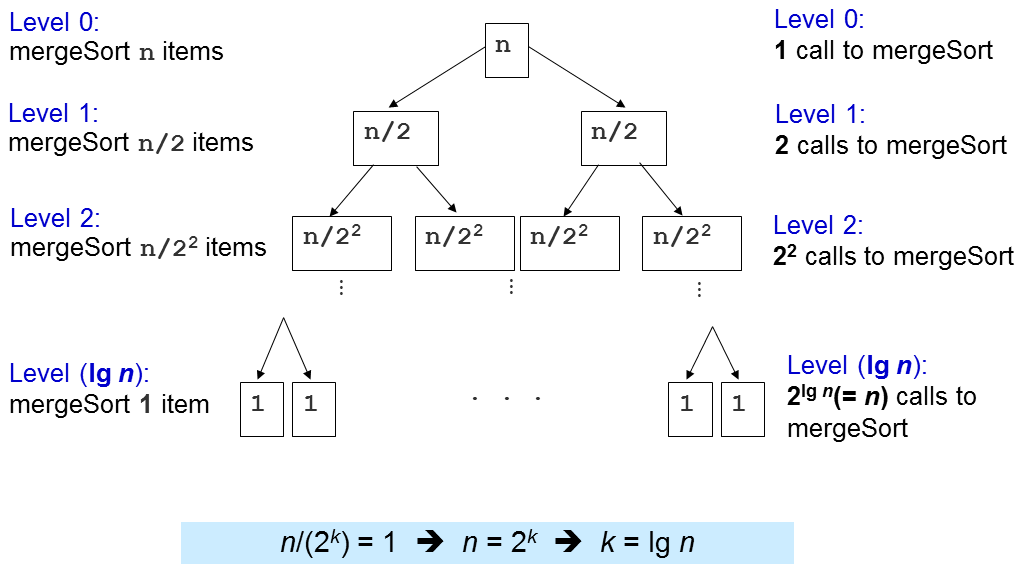X Esc

X Esc

X Esc

X Esc

X Esc

We will dissect this Quick Sort algorithm by first discussing its most important sub-routine: The O(N) partition (classic version).

To partition a[i..j], we first choose a[i] as the pivot p.

The remaining items (i.e. a[i+1..j]) are divided into 3 regions:

1. S1 = a[i+1..m] where items are < p,
2. S2 = a[m+1..k-1] where items are ≥ p, and
3. Unknown = a[k..j], where items are yet to be assigned to either S1 or S2.

Discussion: Why do we choose p = a[i]? Are there other choices?

Harder Discussion: Is it good to always put item(s) that is/are == p on S2 at all times?

X Esc

e-Lecture: The content of this slide is hidden and only available for legitimate CS lecturer worldwide. Drop an email to visualgo.info at gmail dot com if you want to activate this CS lecturer-only feature and you are really a CS lecturer (show your University staff profile).

X Esc

1. 如果 a [k]≥p，则将 a[k] 放入 S2
2. 否则，将 a[k] 放入 S1 中。

X Esc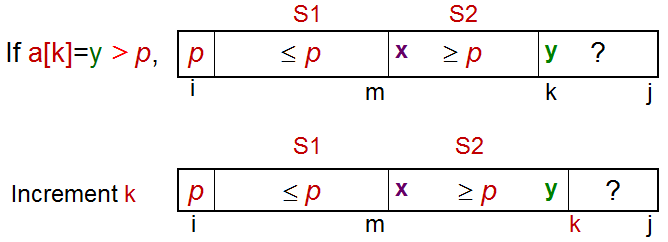X Esc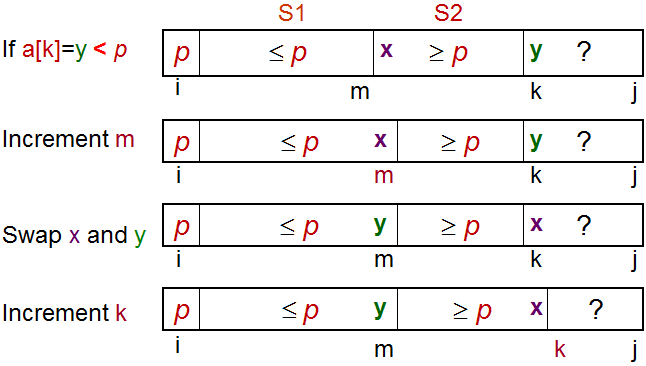X Esc

`int partition(int a[], int i, int j) {  int p = a[i]; // p 是枢纽  int m = i; // S1 和 S2 一开始是空的  for (int k = i+1; k <= j; k++) { // 探索未知的区域    if (a[k] < p) { // case 2      m++;      swap(a[k], a[m]); // C++ STL algorithm std::swap    } // 注意：在情况1的时候我们什么不做: a[k] >= p  }  swap(a[i], a[m]); // 最后一步, 用a[m]交换枢纽  return m; // 返回枢纽的指数, 用于快速排序（Quick Sort）}`
X Esc

`void quickSort(int a[], int low, int high) {  if (low < high) {    int m = partition(a, low, high); // O(N)    // a[low..high] ~> a[low..m–1], pivot, a[m+1..high]    quickSort(a, low, m-1); // 递归地将左子阵列排序    // a[m] = pivot 在分区后就被排序好了    quickSort(a, m+1, high); // 然后将右子阵列排序  }}`
X Esc

X Esc

X Esc

X Esc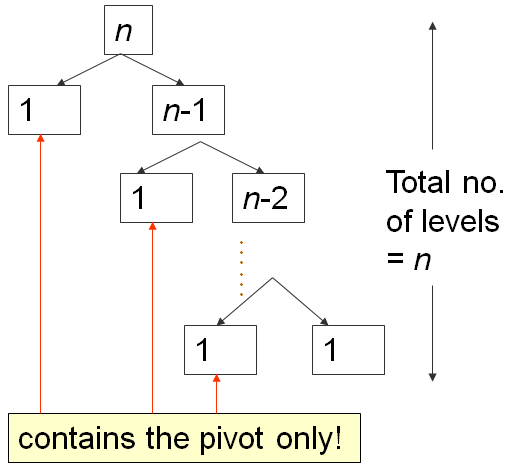第一个分区需要O（N）时间，将a分成0,1，N-1个项目，然后向右递归。

X Esc

X Esc

X Esc

X Esc

1. 计数排序,
2. 基数排序.

X Esc

X Esc

X Esc

X Esc

e-Lecture: The content of this slide is hidden and only available for legitimate CS lecturer worldwide. Drop an email to visualgo.info at gmail dot com if you want to activate this CS lecturer-only feature and you are really a CS lecturer (show your University staff profile).

X Esc

X Esc

X Esc

X Esc

e-Lecture: The content of this slide is hidden and only available for legitimate CS lecturer worldwide. Drop an email to visualgo.info at gmail dot com if you want to activate this CS lecturer-only feature and you are really a CS lecturer (show your University staff profile).

X Esc

X Esc

Quiz: Which of these algorithms run in O(N log N) on any input array of size N?

Quick Sort (Deterministic)
Bubble Sort
Insertion Sort
Merge Sort
X Esc

Quiz: Which of these algorithms has worst case time complexity of Θ(N^2) for sorting N integers?

Insertion Sort
Selection Sort
Merge Sort
Bubble Sort
Θ是一个紧密的时间复杂度分析，其中最佳情况Ω和最坏情况的big-O 分析互相匹配。
X Esc

X Esc

e-Lecture: The content of this slide is hidden and only available for legitimate CS lecturer worldwide. Drop an email to visualgo.info at gmail dot com if you want to activate this CS lecturer-only feature and you are really a CS lecturer (show your University staff profile).

X Esc

e-Lecture: The content of this slide is hidden and only available for legitimate CS lecturer worldwide. Drop an email to visualgo.info at gmail dot com if you want to activate this CS lecturer-only feature and you are really a CS lecturer (show your University staff profile).

X Esc

Actually, the C++ source code for many of these basic sorting algorithms are already scattered throughout these e-Lecture slides. For other programming languages, you can translate the given C++ source code to the other programming language.

Usually, sorting is just a small part in problem solving process and nowadays, most of programming languages have their own sorting functions so we don't really have to re-code them unless absolutely necessary.

In C++, you can use std::sort, std::stable_sort, or std::partial_sort in STL algorithm.
In Java, you can use Collections.sort.
In Python, you can use sort.
In OCaml, you can use List.sort compare list_name.

If the comparison function is problem-specific, we may need to supply additional comparison function to those built-in sorting routines.

X Esc

X Esc

Kattis - mjehuric
Kattis - sortofsorting，或者
Kattis - sidewayssorting

X Esc

X Esc

e-Lecture: The content of this slide is hidden and only available for legitimate CS lecturer worldwide. Drop an email to visualgo.info at gmail dot com if you want to activate this CS lecturer-only feature and you are really a CS lecturer (show your University staff profile).

X Esc

-／+：减缓／增加速度

X Esc

Note that if you notice any bug in this visualization or if you want to request for a new visualization feature, do not hesitate to drop an email to the project leader: Dr Steven Halim via his email address: stevenhalim at gmail dot com.

X Esc

0 1 2 3 4 5 6 7 8 9#### 关于

VisuAlgo在2011年由Steven Halim博士概念化，作为一个工具，帮助他的学生更好地理解数据结构和算法，让他们自己和自己的步伐学习基础。
VisuAlgo包含许多高级算法，这些算法在Steven Halim博士的书（“竞争规划”，与他的兄弟Felix Halim博士合作）和其他书中讨论。今天，一些高级算法的可视化/动画只能在VisuAlgo中找到。

VisuAlgo不是从一开始就设计为在小触摸屏（例如智能手机）上工作良好，因为需要满足许多复杂的算法可视化，需要大量的像素和点击并拖动手势进行交互。一个令人尊敬的用户体验的最低屏幕分辨率为1024x768，并且只有着陆页相对适合移动设备。
VisuAlgo是一个正在进行的项目，更复杂的可视化仍在开发中。

zh, id, kr, vn, th.

#### 团队

Dr Steven Halim, Senior Lecturer, School of Computing (SoC), National University of Singapore (NUS)
Dr Felix Halim, Software Engineer, Google (Mountain View)

Koh Zi Chun, Victor Loh Bo Huai

Phan Thi Quynh Trang, Peter Phandi, Albert Millardo Tjindradinata, Nguyen Hoang Duy

Rose Marie Tan Zhao Yun, Ivan Reinaldo

Jonathan Irvin Gunawan, Nathan Azaria, Ian Leow Tze Wei, Nguyen Viet Dung, Nguyen Khac Tung, Steven Kester Yuwono, Cao Shengze, Mohan Jishnu

Erin Teo Yi Ling, Wang Zi

Truong Ngoc Khanh, John Kevin Tjahjadi, Gabriella Michelle, Muhammad Rais Fathin Mudzakir

List of translators who have contributed ≥100 translations can be found at statistics page.